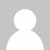LINQ’s `Sum` method is great for calculating a total from all elements in a sequence. But what if you want to track a running total? Well, that’s where the `Scan` method from the MoreLINQ NuGet package comes in really handy. We need to provide a “seed” value for the “accumulator” or “state”, which will be 0 in our case, and a method that calculates a new value based on the current element and the value of the accumulator. In our case, we just need to add the current value to the running total:

``````(new int[] { 4, 1, 2, 6, 7, 2, 5 })
.Scan(0, (acc,next) => acc+next)
``````

If we run this we get a sequence of the following values: 0,4,5,7,13 and so on. Notice that the output sequence includes the seed value, so the output sequence will contain one more element than the input.

There is actually another overload that doesn’t take a seed. It can deduce that the seed is 0 as it is the default value for an int. If we call this version though, we won’t get the initial 0 in the output sequence.

``````(new int[] { 4, 1, 2, 6, 7, 2, 5 })
.Scan((acc,next) => acc+next)
``````

Let’s look at a slightly more complex example, inspired by a programming puzzle I attempted recently.

Suppose we have a sequence of directions (up, down, left, right), represented as characters in a string, and we want to track the current position in x and y coordinates. This requires us to pass through a “state” representing the current position. So here, we simply use a `Tuple<int,int>` to represent the current position, passing in a seed value of (0,0) and then for each element we return a new tuple with the position updated.

We can then use another handy MoreLinq extension method, `ToDelimitedString` to turn the output into a simple string representing the positions.

``````">>^^<v<v"
.Scan(Tuple.Create(0,0), (acc,dir) =>
(dir == '>') ? Tuple.Create(acc.Item1+1,acc.Item2) :
(dir == '^') ? Tuple.Create(acc.Item1,acc.Item2+1) :
(dir == '<') ? Tuple.Create(acc.Item1-1,acc.Item2) :
Tuple.Create(acc.Item1,acc.Item2-1))
.Select(p => \$"({p.Item1},{p.Item2})")
.ToDelimitedString(",")
``````

When we run this, we get the sequence of current positions:

``````(0,0),(1,0),(2,0),(2,1),(2,2),(1,2),(1,1),(0,1),(0,0)
``````

By the way, if you’re an F# developer, then you have a built in Seq.scan function you can use to achieve the same thing.

Want to learn more about LINQ? Be sure to check out my Pluralsight course LINQ Best Practices.